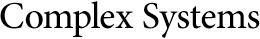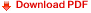## Continuous-valued Cellular Automata for Nonlinear Wave EquationsDaniel N. Ostrov
Department of Mathematics,
Santa Clara University,
Santa Clara, CA 95053

Rudy Rucker
Department of Mathematics and Computer Science,
San Jose State University,
San Jose CA, 95192

#### Abstract

In 1955, E. Fermi, J. Pasta, and S. Ulam investigated quadratic and cubic schemes for numerically simulating nonlinear waves. We derive the partial differential equations corresponding to the Fermi--Pasta--Ulam schemes, present a discussion of the accuracy and stability of different schemes for the equations, and implement the schemes as continuous-valued celluar automata (CA). Some illustrative runs of CAPOW, a CA simulator, are presented which demonstrate the behavior of the different schemes both in stable and unstable domains. These runs include a confirmation of an observation in  regarding the approximate temporal periodicity of the quadratic and cubic nonlinear wave equations.